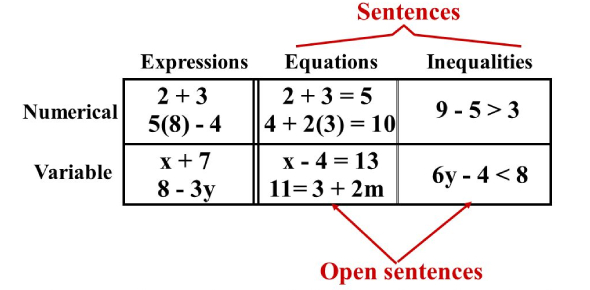# Expressions And Equations Quiz

Approved & Edited by ProProfs Editorial Team
The editorial team at ProProfs Quizzes consists of a select group of subject experts, trivia writers, and quiz masters who have authored over 10,000 quizzes taken by more than 100 million users. This team includes our in-house seasoned quiz moderators and subject matter experts. Our editorial experts, spread across the world, are rigorously trained using our comprehensive guidelines to ensure that you receive the highest quality quizzes.
| By Msmortonoasis
M
Msmortonoasis
Community Contributor
Quizzes Created: 1 | Total Attempts: 3,120
Questions: 11 | Attempts: 3,133SettingsAnswer the questions on this quiz. Do not use your book, workbook, or homework. You may use paper to help you. A parent or other "grown-up" needs to sign their name on the last question to prove you did your own work.

• 1.

### What does 25k mean?

• A.

• B.

The difference between k and 25

• C.

The product of 25 and k

• D.

The product of 2 and 5 and k

C. The product of 25 and k
Explanation
The correct answer is "the product of 25 and k". This means that 25k is the result of multiplying 25 and k together.

Rate this question:

• 2.

### If m = 17, what is the sum of 5 and m?

• A.

85

• B.

22

• C.

21

• D.

84

B. 22
Explanation
The question asks for the sum of 5 and m, where m is given as 17. To find the sum, we add 5 and 17, which equals 22. Therefore, the correct answer is 22.

Rate this question:

• 3.

### In an input-output (function) machine, I put in 5 and get 10. I put in 9 and get 14. I put in 13 and get 18. What is the rule?

• A.

Multiply by 2

• B.

• C.

• D.

Explanation
The rule for the input-output machine is to add 5 to the input number in order to get the output number. This can be observed by the pattern where each input number is increased by 5 to obtain the corresponding output number.

Rate this question:

• 4.

### Evaluate x + 9.2 = 27.4

18.2
Explanation
To evaluate the equation x + 9.2 = 27.4, we can start by subtracting 9.2 from both sides of the equation. This will give us x = 18.2. Therefore, the value of x that satisfies the equation is 18.2.

Rate this question:

• 5.

### If e = 2, what is 8 divided by e?

• A.

4

• B.

10

• C.

6

• D.

28

A. 4
Explanation
If e = 2, then dividing 8 by e would be equivalent to dividing 8 by 2, which equals 4.

Rate this question:

• 6.

### If the rule for the input/output (function) is 25 - g, what is the answer if I put in 19?

6
Explanation
The given rule states that the output is obtained by subtracting the input value from 25. Therefore, if we input 19 into the function, we subtract 19 from 25, resulting in an output of 6.

Rate this question:

• 7.

### What is the algebraic expression for "a number f decreased by 18"?

• A.

18f

• B.

F + 18

• C.

F - 18

• D.

18 + f

C. F - 18
Explanation
The algebraic expression for "a number f decreased by 18" is f - 18. This is because when we say "decreased by," it implies subtraction. So, we subtract 18 from the number f to represent the decrease.

Rate this question:

• 8.

### Solve the equation 100p = 500

• A.

50

• B.

P = 50

• C.

5

• D.

P = 5

D. P = 5
Explanation
The equation is solved by dividing both sides by 100 to isolate the variable p. This results in p = 500/100, which simplifies to p = 5.

Rate this question:

• 9.

### W = 7.8 so what is 29.2 - w ?

• A.

22.4

• B.

22.6

• C.

21.4

• D.

21.6

C. 21.4
Explanation
The given question asks for the result of subtracting the value of w (7.8) from 29.2. By performing the subtraction, we get 29.2 - 7.8 = 21.4. Therefore, the correct answer is 21.4.

Rate this question:

• 10.

### What is the difference between an expression and an equation?

• A.

They are the same thing.

• B.

An equation has an equal sign, but an expression does not.

• C.

An equation has more numbers and an expression has more variables.

• D.

An expression always has a higher value for the variable.

B. An equation has an equal sign, but an expression does not.
Explanation
An equation is a mathematical statement that shows that two expressions are equal, and it is represented by an equal sign. On the other hand, an expression is a mathematical phrase that may contain variables, numbers, and operations, but it does not have an equal sign. Therefore, the correct answer is that an equation has an equal sign, but an expression does not.

Rate this question:

• 11.Back to top